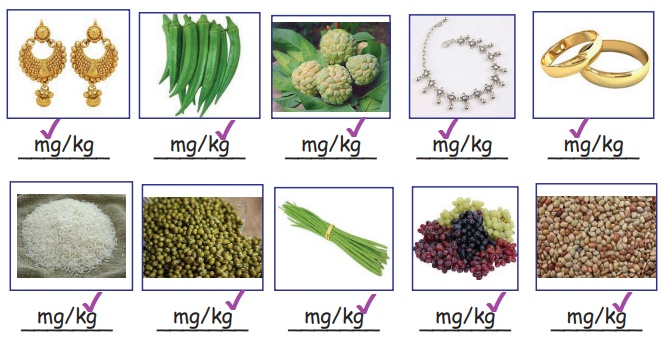Home | | Maths 5th Std | Weight

# Weight

Able to apply the four operations in solving problems involving weight.

UNIT−4

MeasurementsWEIGHT

Able to apply the four operations in solving problems involving weight.

Recall

Sort out the following things and writeLet us recall

10 milligram = 1 centigram

10 centigram = 1 decigram

10 decigram = 1 gram

10 gram = 1 decagram

10 decagram = 1 hectagram

10 hectagram = 1 kilogram

Weighing Machines:

These are some examples of weighing machinesDo you know?

1 gram = 1000 milligram

1 kilogram = 1000 gram

½ kilogram = 500 gram

¼ kilogram = 250 gram

¾ kilogram = 750 gram

Activity

Tick the suitable unit to measure the following objectTags : Measurements | Term 2 Chapter 4 | 5th Maths , 5th Maths : Term 2 Unit 4 : Measurements
Study Material, Lecturing Notes, Assignment, Reference, Wiki description explanation, brief detail
5th Maths : Term 2 Unit 4 : Measurements : Weight | Measurements | Term 2 Chapter 4 | 5th Maths